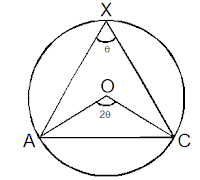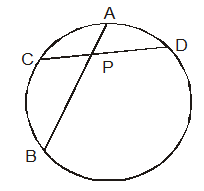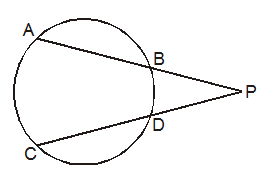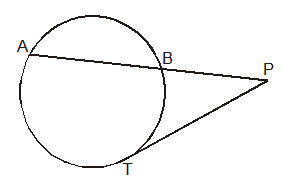# Circles

Important Points:

1. Perpendicular drawn to the chord always divides the chord into two equal parts2. A chord makes an angle $\theta$ on the circumference, it always makes double the angle (2$\theta$) at the center.3. Two tangents drawn to a circle from an external point P are always equal.  Also the circle radius makes right angle with the tangent.4. The angle made by a chord and tangent to the circle is equal to the angle made by the chord in the alternate segment. This is called the alternate segment theorem.In this diagram, AP is a chord whcih makes angle x with the tangent and angle x in the alternate segment in the circle.
$\angle APD = \angle ABP$ and $\angle APC = \angle AEP$

5. If two chords AB and CD intersect each other internally, thenAP x PB = CP x PD

6.  If two line segments (extended chords) PA and PC intersect each other externally, thenPA × PB = PC × PD

7.  If PBA is a secant and PT is a tangent segment, then$PA \times PB = P{T^2}$

8. A chord will make equal angles on the circumference